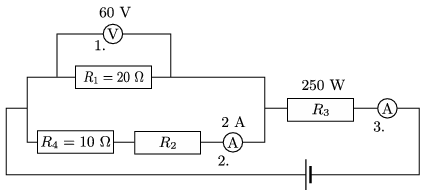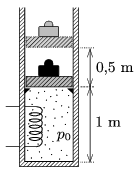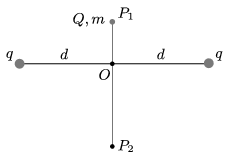Mathematical and Physical Journal
for High Schools
Issued by the MATFUND Foundation
 Already signed up? New to KöMaL?

# KöMaL Problems in Physics, September 2016

Show/hide problems of signs:## Problems with sign 'M'

Deadline expired on October 10, 2016.

M. 361. Let us hang objects of different mass to the the same spring one after the other. (The mass of each object should be greater than that of the spring.) Measure the periods of the oscillations, and with the help of the data determine the mass of the spring reduced to the oscillation''. According to the measurement what percent of the real mass of the spring is the measured reduced mass?

(The mass reduced to the oscillation is that mass $\displaystyle m^*$ which should be added to the mass of the oscillating object $\displaystyle m$, such that the following formula holds true: $\displaystyle T=2\pi\sqrt{\frac{m+m^*}{D}}$.)

(6 pont)

statistics## Problems with sign 'G'

Deadline expired on October 10, 2016.

G. 578. A dog is running along a long straight road wagging its tail. Is it possible that the average speed of the end of its tail is less than the average speed of the centre of mass of the dog?

(3 pont)

solution (in Hungarian), statistics

G. 579. The circuit shown in the figure was assembled.$\displaystyle a)$ What are the resistances of the resistors $\displaystyle R_2$ and $\displaystyle R_3$?

$\displaystyle b)$ What is the reading on meter 3?

$\displaystyle c)$ What is the voltage and the dissipated power of the voltage supply?

(3 pont)

solution (in Hungarian), statistics

G. 580. How much $\displaystyle 0~{}^\circ \rm C$ ice must be put into 1.5 litres of water at a temperature of $\displaystyle 40~{}^\circ \rm C$, in order that the ice melts and the water cools down to the temperature of $\displaystyle 0~{}^\circ \rm C$?

(3 pont)

solution (in Hungarian), statistics## Problems with sign 'P'

Deadline expired on October 10, 2016.

P. 4856. A heavy object is projected upward at an initial speed of $\displaystyle v_0$. What should the initial speed of another object projected upward $\displaystyle \Delta t$ time later be in order that the two objects meet while the first one

$\displaystyle a)$ is still ascending;

$\displaystyle b)$ is at the highest point;

$\displaystyle c)$ is descending?

$\displaystyle d)$ What should the initial speed of the second object be in order that the descending first object meets the second one while the second one is ascending, is stationary, or is descending?

Data: $\displaystyle v_0=5.0$ m/s, $\displaystyle \Delta t=0.3$ s. (Air drag is negligible.)

(4 pont)

solution (in Hungarian), statistics

P. 4857. A uniform rod is hung at one end by means of a hinge. The rod is displaced to a horizontal position and then released.

$\displaystyle a)$ Which will pass the vertical position first: the rod or a simple pendulum which has the same length as the rod?

$\displaystyle b)$ At which position of the swinging rod will the direction of the acceleration of each moving point of the rod be horizontal?

Friction and air drag are negligible.

(5 pont)

solution (in Hungarian), statistics

P. 4858. There is a thermally insulated loaded piston of total mass 1 ton (with the load) in a thermally insulated cylinder of cross sectional area 0.5 m$\displaystyle {}^2$. The piston is supported by a bumper at a height of 1 m measured from the bottom of the cylinder. The load and the piston should be raised by 0.5 metres such that the air in the closed chamber is heated by an electric heater of power rating 1000 W. Initially both the inside pressure and ambient air pressure are $\displaystyle 10^5$ Pa, and the temperature is 300 K.$\displaystyle a)$ For how long should the heater be operated in order to raise the load?

$\displaystyle b)$ What percent of the added heat is used for raising the piston?

(4 pont)

solution (in Hungarian), statistics

P. 4859. A straight wire, having a certain length, is attached to a rechargeable battery of small internal resistance. The wire warms up to a temperature of $\displaystyle 37~{}^\circ$C, while the ambient temperature is $\displaystyle 27~{}^\circ$C. Then one third of the wire is cut off and the remaining part is attached to the same rechargeable battery. What will the eventual temperature of the wire be?

(4 pont)

solution (in Hungarian), statistics

P. 4860. Two fixed, point-like charges of charge $\displaystyle q$ are found at the endpoints of a horizontal line segment of length $\displaystyle 2d$. A ball of mass $\displaystyle m$ and of charge $\displaystyle Q$ is dropped from point $\displaystyle P_1$ which is on the vertical, perpendicular bisector of the line segment. The ball stops exactly at point $\displaystyle P_2$.$\displaystyle a)$ What is the mass of the ball?

$\displaystyle b)$ What is the acceleration of the ball at point $\displaystyle P_2$?

Data: $\displaystyle q=2\cdot 10^{-6}$ C, $\displaystyle Q=-6\cdot 10^{-6}$ C, $\displaystyle d=12$ cm, $\displaystyle OP_1=5$ cm, $\displaystyle OP_2=10$ cm.

(4 pont)

solution (in Hungarian), statistics

P. 4861. When there is new moon, a little later after sunset, the dark side of the Moon can also be seen faintly. Why?

(3 pont)

solution (in Hungarian), statistics

P. 4862. When the nucleus of a radium-226, which is at rest, decays, an $\displaystyle \alpha$ particle and the nucleus of radon are created. The released energy during the decay is 4.87 MeV, which appears as the kinetic energy of the two resulted particles. Calculate the linear momentum and the kinetic energy of both the $\displaystyle \alpha$ particle and the radon nucleus.

(4 pont)

solution (in Hungarian), statistics

P. 4863. The density of electric charge on the surface of a plastic sphere of diameter 10 cm is $\displaystyle 10~\mu{\rm C/m}^2$. The sphere is rotated about one of its diameter at a number of revolutions of 10 s$\displaystyle {}^{-1}$. Determine both the direction and the magnitude of the magnetic induction at the centre of the sphere.

(6 pont)

solution (in Hungarian), statistics